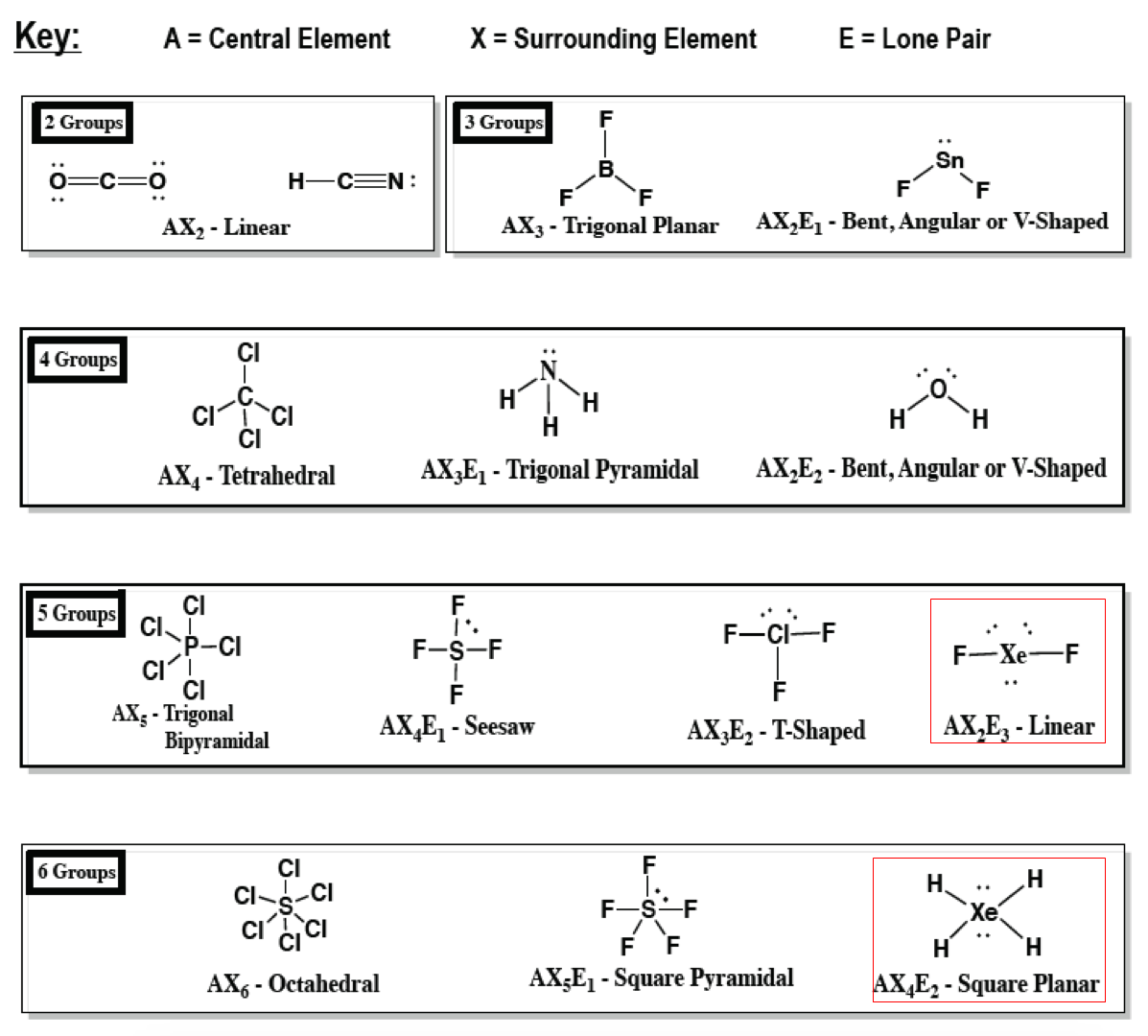# Problem: As a general rule, MXn molecules (where M represents a central atom and X represents terminal atoms; n = 2 – 5) are polar if there is one or more lone pairs of electrons on M. NH3 (M = N, X = H, n = 3) is an example. There are two molecular structures with lone pairs that are exceptions to this rule. What are they?

###### FREE Expert Solution

Exception to polar MXrule: molecules having a symmetrical shape → dipole moments cancel out80% (188 ratings)###### Problem Details

As a general rule, MXn molecules (where M represents a central atom and X represents terminal atoms; n = 2 – 5) are polar if there is one or more lone pairs of electrons on M. NH3 (M = N, X = H, n = 3) is an example. There are two molecular structures with lone pairs that are exceptions to this rule. What are they?

Frequently Asked Questions

What scientific concept do you need to know in order to solve this problem?

Our tutors have indicated that to solve this problem you will need to apply the Molecular Polarity concept. You can view video lessons to learn Molecular Polarity. Or if you need more Molecular Polarity practice, you can also practice Molecular Polarity practice problems.

What professor is this problem relevant for?

Based on our data, we think this problem is relevant for Professor Du's class at UGA.

What textbook is this problem found in?

Our data indicates that this problem or a close variation was asked in Chemistry - OpenStax 2015th Edition. You can also practice Chemistry - OpenStax 2015th Edition practice problems.